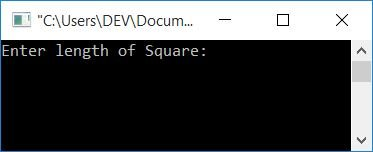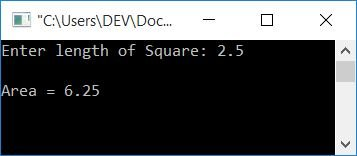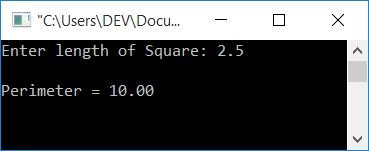# C program to calculate the area and perimeter of a square

In this article, you will learn and get code for the program given below:

#### How to Find the Area of a Square

To find the area of a square, use the simple formula area = len*len or area = len2. len denotes the length of a square's side. All four sides of a square, as we all know, are the same length. Now let's go through the program given below.

## Area of the Square Program in C

To calculate the area of a square in C programming, you have to ask the user to enter the side length of the square and then perform the simple operation of multiplication of the given number with itself (the length of the square), as shown here in the following program:

```#include<stdio.h>
#include<conio.h>
int main()
{
float len, area;
printf("Enter length of Square: ");
scanf("%f", &len);
area = len*len;
printf("\nArea = %0.2f", area);
getch();
return 0;
}```

Here is a sample run of the above program. This is a snapshot of the initial output:Enter the length of the square, say 2.5, and press the ENTER key to see the area as shown in the snapshot of the final output given below:#### Steps used in the above program

Here is a list of some of the main steps used in the above program:

• Declare any two floating-point variables. Because the side length of a square may contain a decimal value,
• Receive the length of the square in the len variable.
• Perform a multiplication operation on the len variable with itself, the initialize it to the area variable.
• Simply print the area value as output.
• The variable area indicates the area of the square on the output screen.
• Here, %0.2f is used to print the value of area up to 2 decimal places only.

## Find the area of a square using a user-defined function in C

Now let's create another program that does the same job as the previous program. Except this program is created using functions. Let's take a look at it:

```#include<stdio.h>
#include<conio.h>
float findAreaSquare(float);
int main()
{
float len, area;
printf("Enter length of Square: ");
scanf("%f", &len);
area = findAreaSquare(len);
printf("\nArea = %0.2f", area);
getch();
return 0;
}
float findAreaSquare(float leng)
{
return leng*leng;
}```

This program produces the same output as the previous program.

#### How to Find the Perimeter of a Square

To find the perimeter of a square, use the formula perimeter = 4*side. Here, side indicates the length of a side of the square. To calculate perimeter, we must first determine the square's boundary length. As a square has four sides of equal length, here we only have to ask for side length and then just multiply it by 4. That will be the perimeter.

## Perimeter of the square program in C

Here is the program in C that calculates the perimeter of a square of given length (by the user at run-time).

```#include<stdio.h>
#include<conio.h>
int main()
{
float len, perimeter;
printf("Enter length of Square: ");
scanf("%f", &len);
perimeter = 4*len;
printf("\nPerimeter = %0.2f", perimeter);
getch();
return 0;
}```

When the above C program is compiled and executed, it will produce the following result:You can clearly understand this program by yourself; nothing extra is included here. So I'm going to the next program, which uses a function to calculate the perimeter of a square.

## Find the perimeter of a square using a user-defined function in C

This is the final program of this article that does the same job as the previous program, that is, asks the user to enter the side length of a square and calculates the perimeter of the square, but this time using a function. Let's have a look at it:

```#include<stdio.h>
#include<conio.h>
float findPeriSquare(float);
int main()
{
float len, perimeter;
printf("Enter length of Square: ");
scanf("%f", &len);
perimeter = findPeriSquare(len);
printf("\nPerimeter = %0.2f", perimeter);
getch();
return 0;
}
float findPeriSquare(float len)
{
return 4*len;
}```

This program produces the same output as the previous program did.

C Quiz

« Previous Program Next Program »